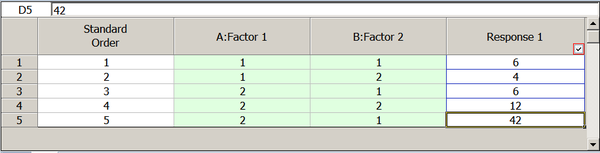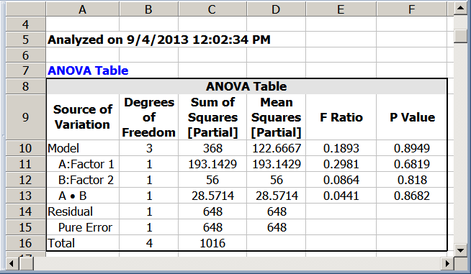# Use of Regression to Calculate Sum of Squares

 Appendix B Use of Regression to Calculate Sum of Squares

This appendix explains the reason behind the use of regression in Weibull++ DOE folios in all calculations related to the sum of squares. A number of textbooks present the method of direct summation to calculate the sum of squares. But this method is only applicable for balanced designs and may give incorrect results for unbalanced designs. For example, the sum of squares for factorin a balanced factorial experiment with two factors,and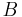, is given as follows:where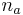represents the levels of factor,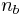represents the levels of factor, andrepresents the number of samples for each combination ofand. The term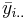is the mean value for the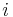th level of factor,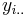is the sum of all observations at theth level of factorand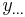is the sum of all observations.

The analogous term to calculate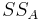in the case of an unbalanced design is given as: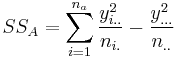whereis the number of observations at theth level of factorand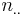is the total number of observations. Similarly, to calculate the sum of squares for factorand interaction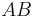, the formulas are given as: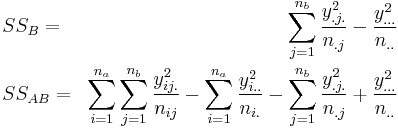Applying these relations to the unbalanced data of the last table, the sum of squares for the interactionis:which is obviously incorrect since the sum of squares cannot be negative. For a detailed discussion on this refer to Searle(1997, 1971).The correct sum of squares can be calculated as shown next. The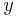and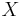matrices for the design of the last table can be written as: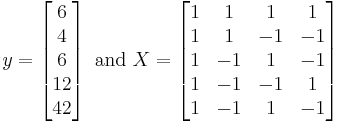Then the sum of squares for the interactioncan be calculated as: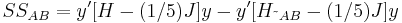where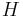is the hat matrix and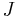is the matrix of ones. The matrix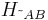can be calculated using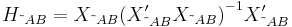where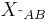is the design matrix,, excluding the last column that represents the interaction effect. Thus, the sum of squares for the interactionis: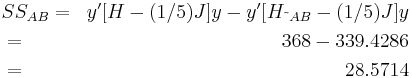This is the value that is calculated by the DOE folio (see the first figure below, for the experiment design and the second figure below for the analysis).